•非线
• 本文主要对简单的连续系统微分方程（含系数矩阵）的MATLAB数值解求法进行归纳，并绘制仿真图像。同时也对一阶和高阶微分方程求法进行总结。 系统方程为一阶积分器的连续系统 目录1. 可解析解的微分方程2. MATLAB...

在多智能体系统（MASs）论文数值仿真时，时常碰到连续系统的情况，其中参数也多为系数矩阵。本文主要对简单的连续系统微分方程（含系数矩阵）的MATLAB数值解求法进行归纳，并绘制仿真图像。同时也对一阶和高阶微分方程求法进行总结。

目录1. 可求解析解的微分方程2. MATLAB函数直接调用3. 其他情形3.1 一阶微分方程3.2 高阶微分方程4. 总结参考资料
1. 可求解析解的微分方程
系统方程为一阶积分器的连续系统 $\dot{x}=u,~~x \in R^n,$
其中为了实现平均一致性，设计控制器  $u=-Lx$, $L \in R^{n \times n}$ 为对应图的拉普拉斯矩阵。
为了进行系统仿真,设 $n=4,L$ 如下，此处连续系统方程为
$\dot{x}==-\begin{pmatrix} 3 & -1 & -1 & -1 \\ -1 & 2 & -1 & 0 \\ -1 & -1 & 3 & -1 \\ -1 & 0 & -1 &2 \\ \end{pmatrix}x$
假设初值 $x_0=(10;0;5;10)$， 对其进行数值求解与仿真。
可见对于如上 $\dot{x}=-Lx$ 形式的微分方程，可用常微分方程的知识，先求出其解析解，再进行数值仿真。解析解为：
$x=e^{-Lt}x_0.$
MATLAB代码如下：
%%初始化设置
step=200;          %定义迭代步数
y=zeros(step,4);   %初始化矩阵来存储迭代值，用于作图
x0=[10; 0; 5; 10]; %微分方程初值
L=[3 -1 -1 -1;     %系数矩阵L
-1  2 -1  0;
-1 -1  3 -1;
-1  0 -1  2];
for i=1:4         %迭代初值
y(1,i)=x(i);
end

%%系统迭代步
for k=2:step      %迭代过程
x=expm(-L*k/50)*x0;   %注意指数矩阵求解用函数expm()
for i=1:4
y(k,i)=x(i);
end
k=k+1;
end

%%仿真作图
t=1:step;
plot(t/50,y(t,1),t/50,y(t,2),t/50,y(t,3),t/50,y(t,4))
xlabel('t');
ylabel('x');
legend('x1','x2','x3','x4')

仿真图像如下：2. MATLAB函数直接调用
在很多情况下，微分方程的解析解是很难求得的，所以才需要求其数值解并进行仿真。这里主要运用MATLAB自带函数ODE45（龙格库塔方法）来求解。
对于题设 1 中问题，我们将其转化为微分方程组，如下：
$\left\{ \begin{array}{c} \dot{x_1}=-[~ 3x_1-x_2-x_3-x_4~] \\ \dot{x_2}=-[~ -x_1+2x_2 -x_3+0~]\\ \dot{x_3}=-[~ -x_1-x_2+3x_3-x_4~] \\ \dot{x_4}=-[~ -x_1+0-x_3+2x_4~] \end{array} \right.$
定义m文件 $func.m$，将函数信息存入此m文件。
function dx=func(t,x)

%% 初始化有4个分量的dx
dx=zeros(4,1);

%% 微分方程
dx(1)=-(3*x(1)-x(2)-x(3)-x(4));    %dx(1)为x第一个分量的导数，下同
dx(2)=-(-x(1)+2*x(2)-x(3)+0);      %x(1)指x的第一个分量，下同
dx(3)=-(-x(1)-x(2)+3*x(3)-x(4));
dx(4)=-(-x(1)+0-x(3)+2*x(4));

如下代码对函数进行调用，并求数值解与仿真。
%% 参数初始化
startt=0;endd=10;      %区间开始和结束
x1=10;x2=0;x3=5;x4=10; %变量初值

%% ode45方法求解数值解
[t,x]=ode45(@func,[startt endd],[x1;x2;x3;x4]);

%% 仿真图像
plot(t,x(:,1),t,x(:,2),t,x(:,3),t,x(:,4))
xlabel('t');
ylabel('x');
legend('x1','x2','x3','x4')

仿真图像绘制如下：3. 其他情形
3.1 一阶微分方程
简单的一阶微分方程，无需将微分方程写入m文件，只需在命令行的ODE45函数中加入微分方程即可。例如求解如下微分方程数值解
$\dot{x}=x+t,$
MATLAB代码如下：
%% 定义x初值
x0=0;
%% ode5求微分方程数值解，其中[0 6]为求解区间
% @(t,x) x+t 为要求解的微分方程表示
[t,x]=ode45(@(t,x) x+t,[0 6],x0);
%% 绘制图像
plot(t,x);

仿真图像显示如下：3.2 高阶微分方程
高阶微分方程求数值解，基本思路是将其化为一阶微分方程组进行求解。例如对于如下二阶微分方程：
$\ddot{x}+x^3+\dot{x}^3=0,\\ x(0)=1,~\dot{x}(0)=0.$
我们将其化为两个一阶微分方程，如下：
$\dot{x}_1=x_2,\\ \dot{x}_2=-{x_1}^3-{x_2}^3.$
其实，$x_1$表示原系统$x$,  $x_2$表示原系统 $\dot{x}$. 化为一阶方程组后，便可采用 2 中介绍的方法，先定义M文件 $funcc.m$ 存储如上微分方程信息。MATLAB代码如下：
function dx=funcc(t,x)
dx=[x(2);
-x(1)^3-x(2)^3];


命令行输入如下代码，调用m文件，并求解与绘图。
%% 给定x1和x2初值
x0=[1;0];

%% 使用ode45求解微分方程
[t,x]=ode45(@funcc,[0 20],x0);

%% 仿真作图（分别绘制了x和x的导函数图像）
plot(t,x(:,1),t,x(:,2))
xlabel('t');
ylabel('x');
legend('x_1','x_2')

仿真图像如下所示：4. 总结

微分方程数值解求法总结：

直接求出其解析解，便可计算其数值解，如情况1。
不可求解析解的形式：
2.1 一阶微分方程，如情况3.1。
2.2 一阶微分方程组，如情况2。
2.3 高阶微分方程，如情况3.2。

参考资料

  https://blog.csdn.net/qq_18820125/article/details/104727013
 https://www.zhihu.com/question/22378594


展开全文ieee论文 matlab 矩阵
• 用牛顿 同伦，参数微分法求非线性方程的根，其中里面有个子程序是牛顿法
• 用系统动力学仿真和微分方程理论方法结合进行反馈供应链的波动规律研究, 首先, 建立沼气产业养殖场至农户反馈供应链三阶基本流图模型, 又建立其仿真方程, 并应用调控参数法, 建立原料供不应求反馈供应链模型, 同时,...
• 参数函数求导 1. 利用莱布尼茨定理高阶导 只看两点： 1、常用导数的高阶公式 2、例题 例题： 2.隐函数求导 这种方程里面y是x的函数，但是不显性。 例题1 设 y=y(x),y2−2xy+9=0y = y(x), y^2 - 2xy +9 = 0y=y(x)...
DAY 4.
这世上总要有个明白人，懂得克制。
文章目录DAY 4.1. 利用莱布尼茨定理求高阶导2.隐函数求导3.对数求导4.参数函数求导5.用导数求切线、法线6.函数的微分
1. 利用莱布尼茨定理求高阶导
只看两点：
1、常用导数的高阶公式
2、例题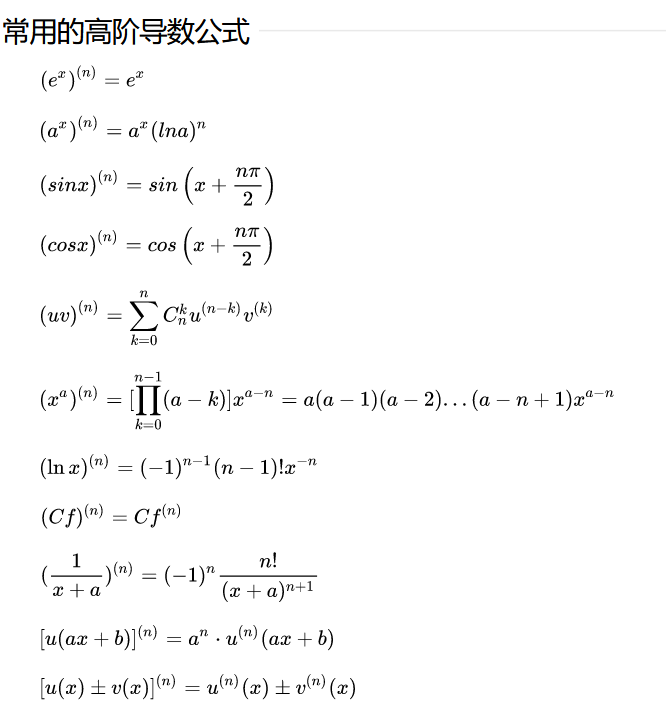例题：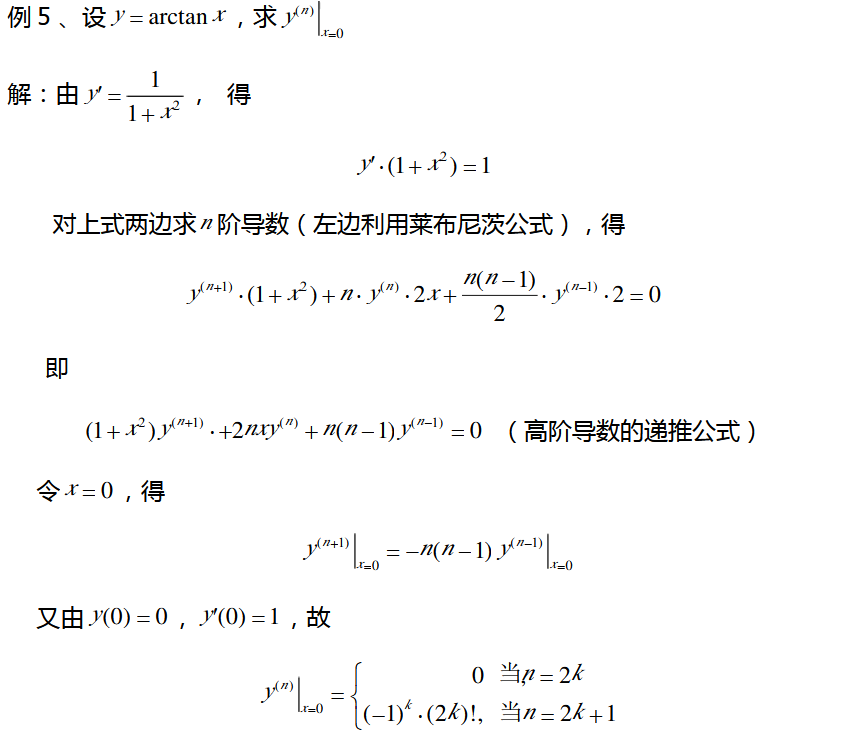2.隐函数求导
这种方程里面y是x的函数，但是不显性。
例题1
设 $y = y(x), y^2 - 2xy +9 = 0$ 求 $\frac{d_y}{d_x}$
解：方程两边同时对x求导得
$2yy' - 2y - 2xy' = 0$
$\frac{d_y}{d_x}$ = $\frac{y}{y - x}$
3.对数求导
例题2
$y = (\frac {x}{1+x}) ^x$ 求 y 的一阶导
解：方程两边同时取对数有
$\ln y = x \ln(\frac {x}{1+x})$
方程两边同时对x求导得
$\frac{y'}{y} = \ln(\frac {x}{1+x}) + x(\frac{1}{x} - \frac{1}{1+x})$
$y' = y(\ln(\frac {x}{1+x}) + 1 - \frac{x}{1+x})$
$y' = (\frac {x}{1+x}) ^x(\ln(\frac {x}{1+x}) +\frac{1}{1+x})$
4.参数函数求导
例题3
$\begin{cases} x = \frac{t^2}{2}& \text{}\\y = 1-t& \text{} \end{cases}$  求 $\frac{d_y}{d_x}$,  $\frac{d^2_y}{d_x{^2}}$
解：
$\frac{d_y}{d_x}$ = $\frac{(1-t)'}{\frac {t^2}{2}'}$ = $\frac { - 1}{t}$
$\frac{d^2_y}{d_x{^2}}$ = $\frac{\frac{-1}{t}'}{\frac {t^2}{2}'}$ = $\frac{1}{t^3}$
5.用导数求切线、法线
这部分的内容和我们在高中学的差不多，基本就是求导数得斜率，再点差法写方程
例题4
求 $y = \cos x$ 在 点$(\frac{\pi}{3}, \frac{1}{2})$ 处的切线与法线方程。
解：对y求导得：
$y = -\sin x$ 代入点得切线的斜率k = $- \frac{\sqrt 3}{2}$
由点差法可得切线的方程为：$y- \frac{1}{2} = - \frac{\sqrt 3}{2}(x -\frac{\pi}{3} )$
而函数的法线方程就只需要将斜率改写为 $\frac{2}{\sqrt 3}$
6.函数的微分
和前面函数的求导一样的只不过要在结尾加上 $d_x$
例题5
已知 $y = x\sin 2x$ ，则 $d_y =$  ___ $d_x$
解：$d_y = (\sin 2x + x \cos 2x * 2$)$d_x$ =  $(\sin 2x + 2x \cos 2x$)$d_x$


展开全文• 关于一元函数微分学，专升本数学考试要求包括：(一)导数与微分1．理解导数的概念及其几何意义，了解左导数与右导数的定义，理解函数的可导性与连续性的关系，会用定义函数在...掌握对数求导参数方程求导。...关于一元函数微分学，专升本数学考试要求包括：(一)导数与微分1．理解导数的概念及其几何意义，了解左导数与右导数的定义，理解函数的可导性与连续性的关系，会用定义求函数在一点处的导数。2．会求曲线上一点处的切线方程与法线方程。3．熟记导数的基本公式，会运用函数的四则运算求导法则，复合函数求导法则和反函数求导法则求导数。会求分段函数的导数。4．会求隐函数的导数。掌握对数求导法与参数方程求导法。5．理解高阶导数的概念，会求一些简单的函数的n阶导数。6．理解函数微分的概念，掌握微分运算法则与一阶微分形式不变性，理解可微与可导的关系，会求函数的一阶微分。(二)中值定理及导数的应用1．理解罗尔(Rolle)中值定理、拉格朗日(Lagrange)中值定理及它们的几何意义，理解柯西(Cauchy)中值定理、泰勒(Taylor)中值定理。会用罗尔中值定理证明方程根的存在性。会用拉格朗日中值定理证明一些简单的不等式。2．掌握洛必达(L’Hospital)法则，会用洛必达法则求未定式的极限。3．会利用导数判定函数的单调性，会求函数的单调区间，会利用函数的单调性证明一些简单的不等式。4．理解函数极值的概念，会求函数的极值和最值，会解决一些简单的应用问题。5．会判定曲线的凹凸性，会求曲线的拐点。6．会求曲线的渐近线(水平渐近线、垂直渐近线和斜渐近线)。7．会描绘一些简单的函数的图形。这一部分，我们来学习对数求导法技巧。对数求导法适用于两类函数，第一类是幂指函数，幂指函数是指具有指数函数形式，且底数和指数都包含未知数x的函数。幂指函数求导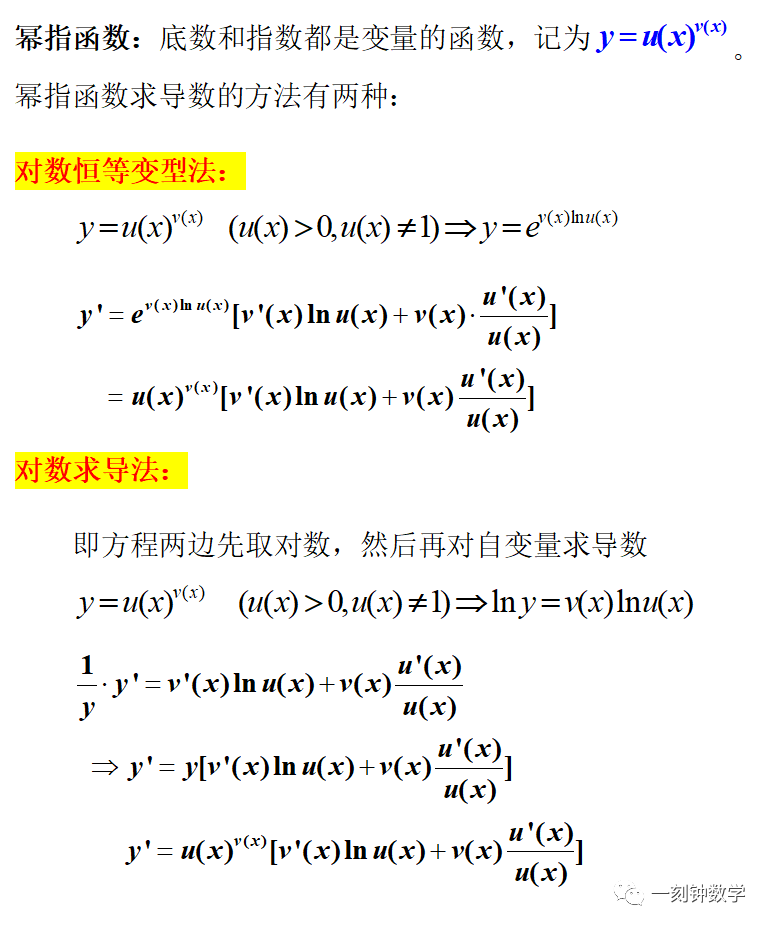典型例题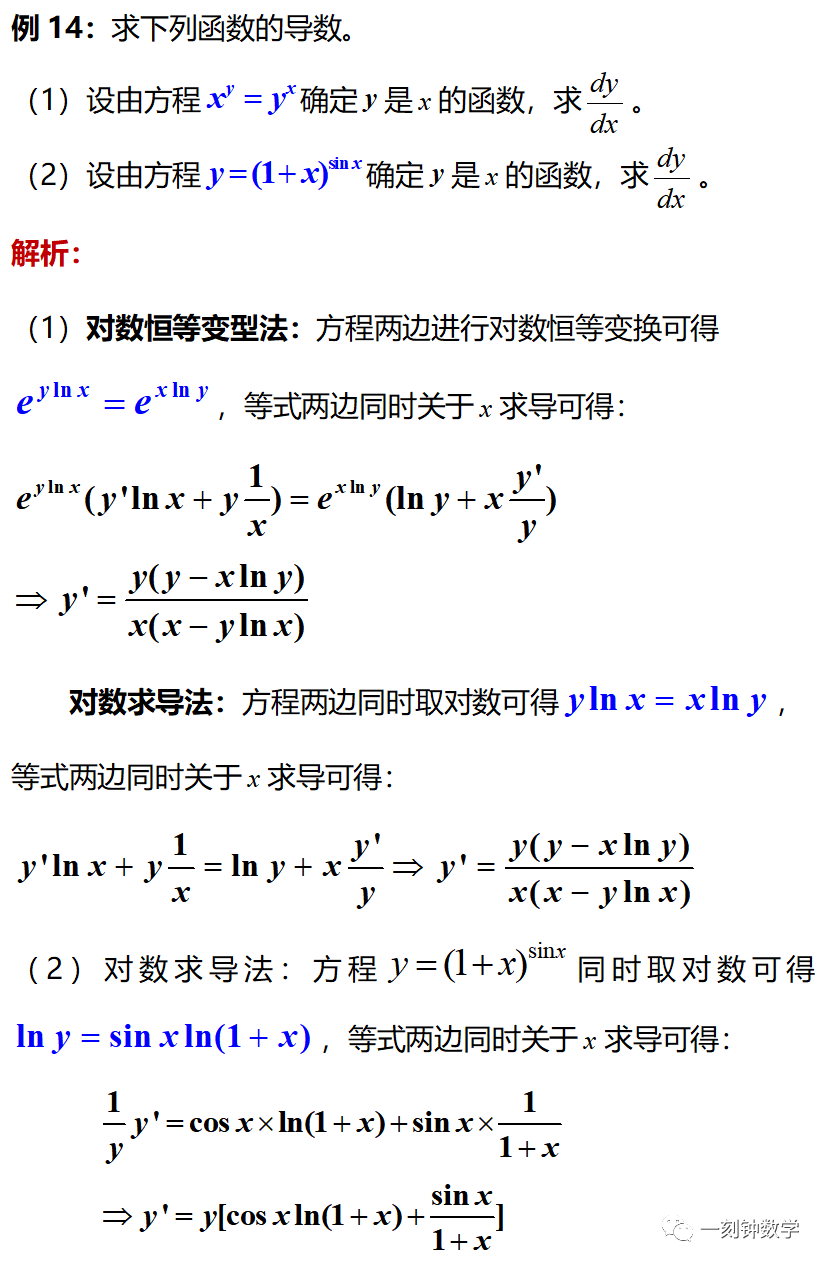注意：对数求导法除了可用于求解幂指函数的导函数之外，还可用于“表达式为若干因子连乘积、乘方、开方或商形的函数”求导，利用对数的性质，“乘法变加法、除法变减法、指数变倍数”，可以大大简化做题的计算量，提高准确率，详见下例。典型例题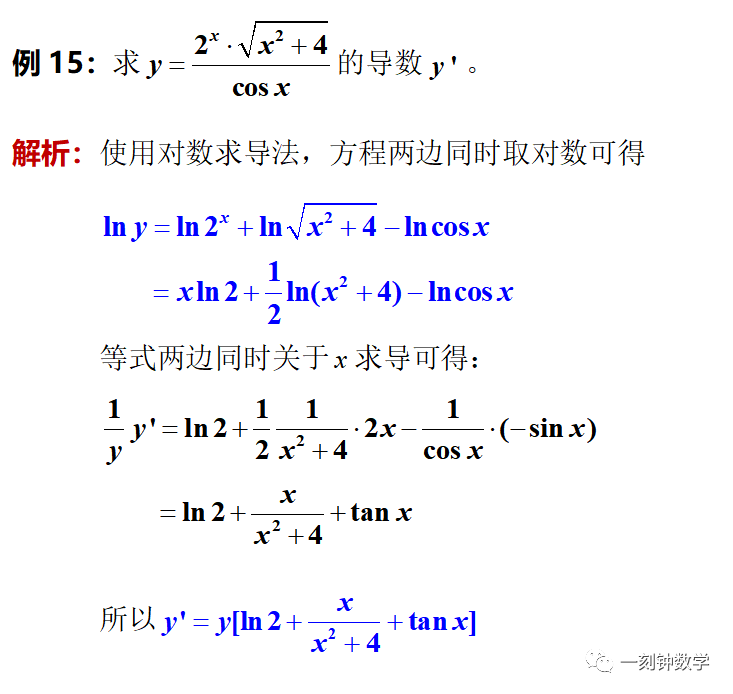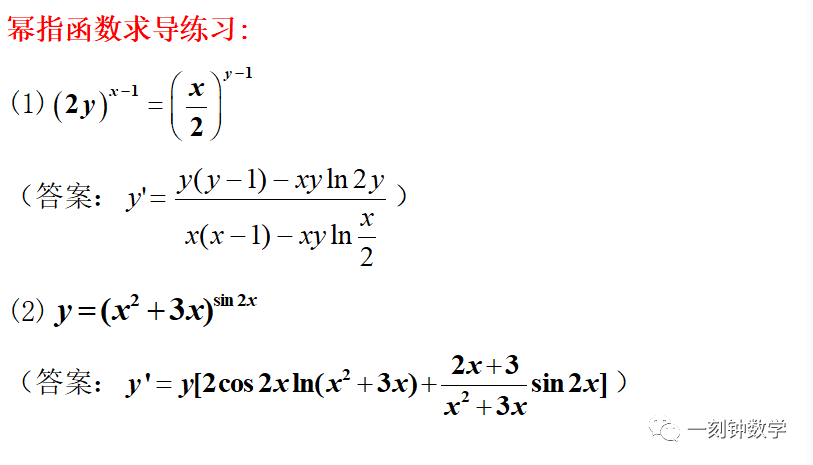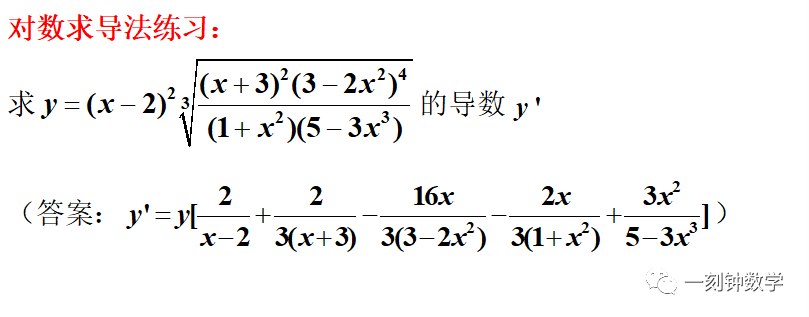展开全文• DiffParam1 用参数微分法中的欧拉法求非线性方程组的一组解 DiffParam2 用参数微分法中的中点积分法求非线性方程组的一组解 mulFastDown 用最速下降法求非线性方程组的一组解 mulGSND 用高斯牛顿法求非线性方程组的...
• DiffParam1 用参数微分法中的欧拉法求非线性方程组的一组解 DiffParam2 用参数微分法中的中点积分法求非线性方程组的一组解 mulFastDown 用最速下降法求非线性方程组的一组解 mulGSND 用高斯牛顿法求非线性方程组的...
• 关于一元函数微分学，专升本数学考试要求包括：(一)导数与微分1．理解导数的概念及其几何意义，了解左导数与右导数的定义，理解函数的可导性与连续性的关系，会用定义函数在...掌握对数求导参数方程求导。...关于一元函数微分学，专升本数学考试要求包括：(一)导数与微分1．理解导数的概念及其几何意义，了解左导数与右导数的定义，理解函数的可导性与连续性的关系，会用定义求函数在一点处的导数。2．会求曲线上一点处的切线方程与法线方程。3．熟记导数的基本公式，会运用函数的四则运算求导法则，复合函数求导法则和反函数求导法则求导数。会求分段函数的导数。4．会求隐函数的导数。掌握对数求导法与参数方程求导法。5．理解高阶导数的概念，会求一些简单的函数的n阶导数。6．理解函数微分的概念，掌握微分运算法则与一阶微分形式不变性，理解可微与可导的关系，会求函数的一阶微分。(二)中值定理及导数的应用1．理解罗尔(Rolle)中值定理、拉格朗日(Lagrange)中值定理及它们的几何意义，理解柯西(Cauchy)中值定理、泰勒(Taylor)中值定理。会用罗尔中值定理证明方程根的存在性。会用拉格朗日中值定理证明一些简单的不等式。2．掌握洛必达(L’Hospital)法则，会用洛必达法则求未定式的极限。3．会利用导数判定函数的单调性，会求函数的单调区间，会利用函数的单调性证明一些简单的不等式。4．理解函数极值的概念，会求函数的极值和最值，会解决一些简单的应用问题。5．会判定曲线的凹凸性，会求曲线的拐点。6．会求曲线的渐近线(水平渐近线、垂直渐近线和斜渐近线)。7．会描绘一些简单的函数的图形。这一部分，我们来学习反函数求导技巧。在此之前，我们先来学习抽象函数求导。解析式(表达式)没有完全给出的函数，可以称为抽象函数。求解这种类型函数的导函数要深刻理解函数的概念、熟练掌握复合函数求导法则。典型例题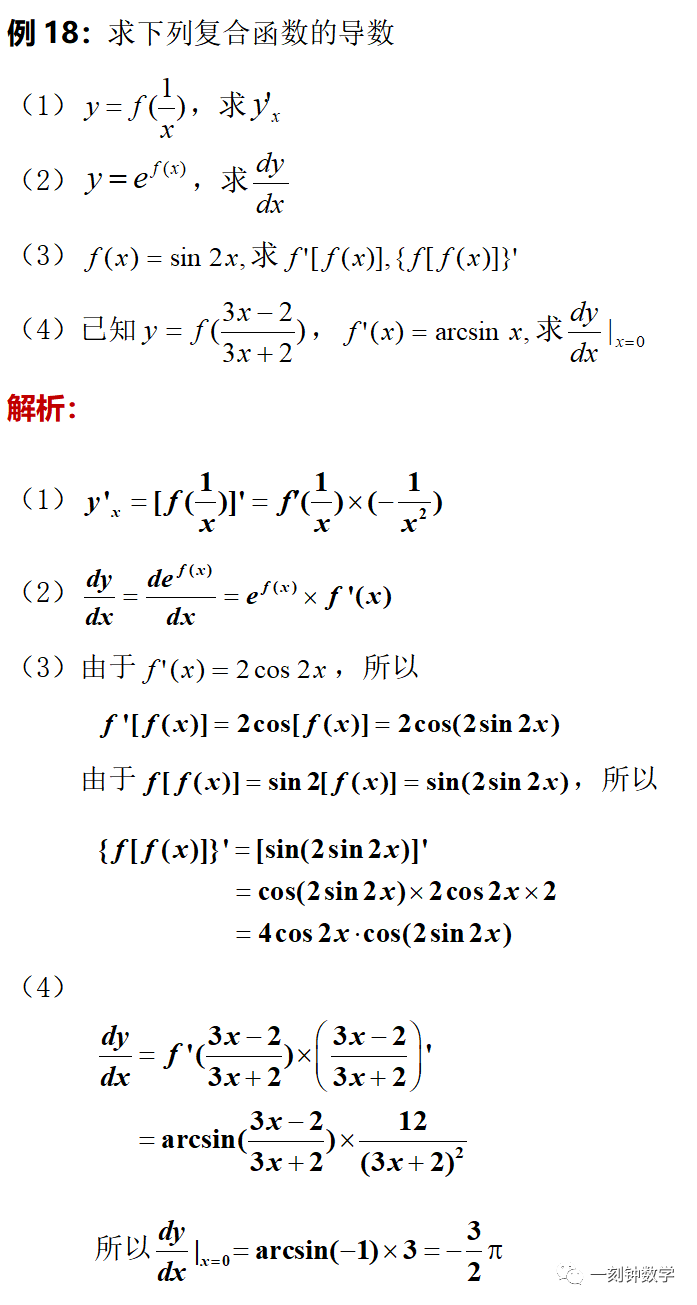掌握了抽象函数求导的方法之后，下面我们来学习反函数求导方法。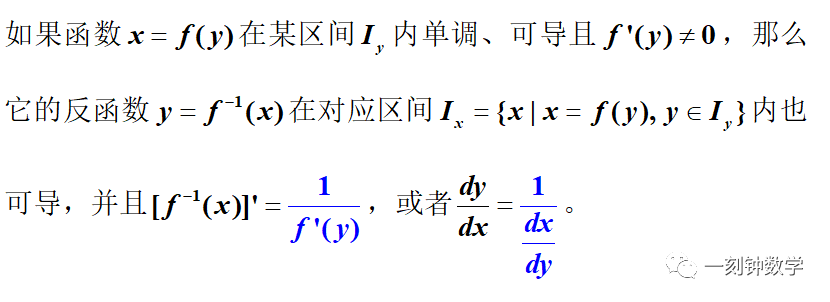对于反函数相关问题，要深刻理解反函数的求解过程以及导数的概念(函数值的变化量比上自变量的变化量)。典型例题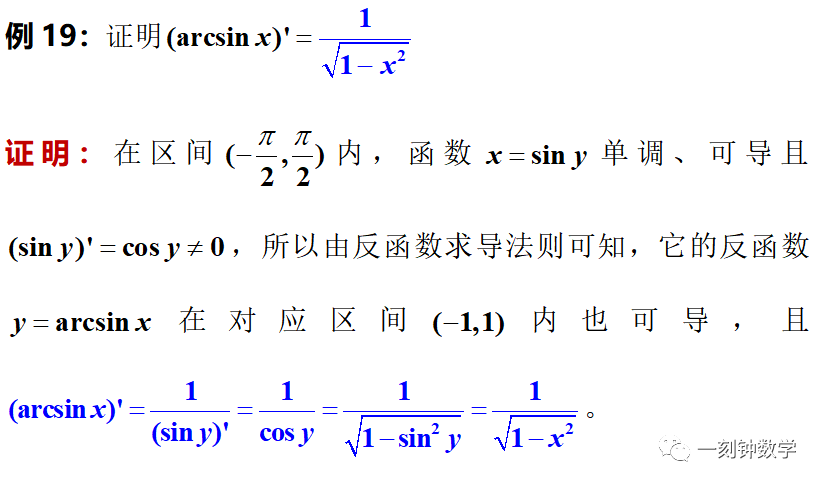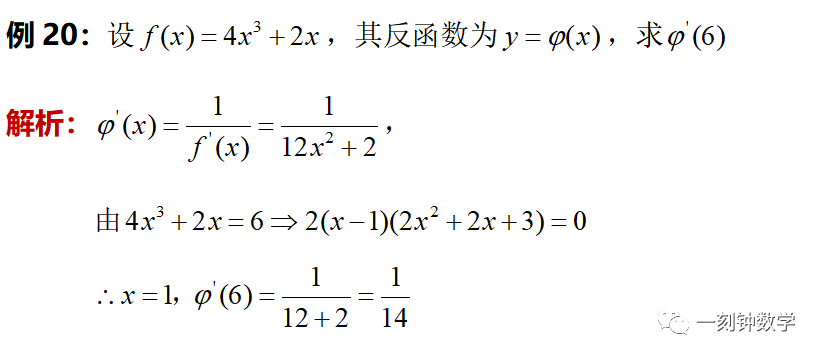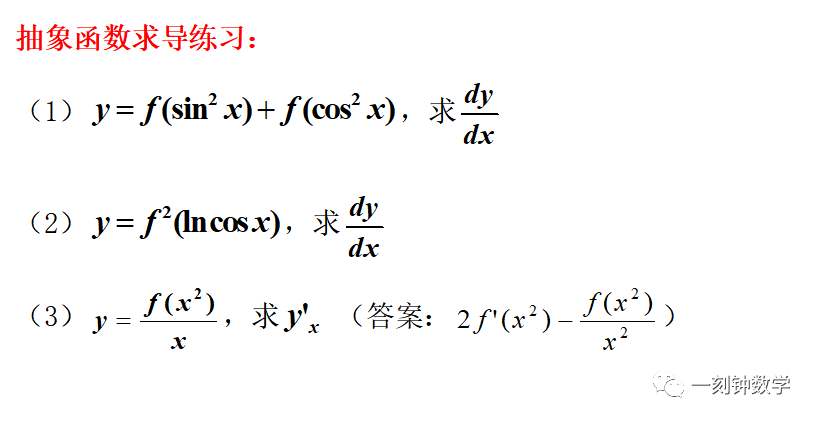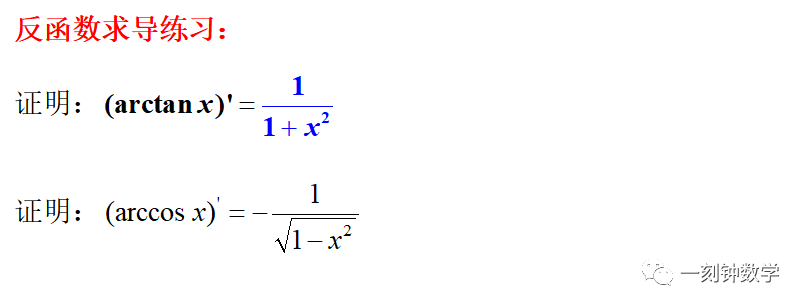END点个赞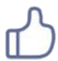再走吧点击查看往期内容02一元函数微分学(占比30%)往期回顾一元函数微分学考点(8)：参数方程求导一元函数微分学考点(7)：对数求导法一元函数微分学考点(6)：隐函数求导一元函数微分学考点(5)：分段函数求导一元函数微分学考点(4)：复合函数求导一元函数微分学考点(3)：导数计算之四则运算一元函数微分学考点(2)：导数的几何意义一元函数微分学考点(1)：导数的概念01函数、极限与连续(占比20%)往期回顾函数、极限与连续：真题讲解函数、极限与连续：未定式总结函数、极限与连续考点(15)：连续函数的性质函数、极限与连续考点(14)：连续与间断函数、极限与连续考点(13)：曲线渐近线函数、极限与连续考点(12)：洛必达求极限函数、极限与连续考点(11)：等价无穷小求极限函数、极限与连续考点(10)：两个重要极限公式函数、极限与连续考点(9)：抓大头法求极限函数、极限与连续考点(8)：有理化法法求极限函数、极限与连续考点(7)：因式分解法求极限函数、极限与连续考点(6)：四则运算求极限函数、极限与连续考点(5)：极限的基本性质函数、极限与连续考点(4)：反函数函数、极限与连续考点(3)：函数的基本性质函数、极限与连续考点(2)：函数的表达式函数、极限与连续考点(1)：函数的定义域专升本数学简介扫码关注·永不迷路一刻钟数学

展开全文• 机器学习的数学基础 ...复合函数，反函数，隐函数以及参数方程所确定的函数的微分法8.常用高阶导数公式9.微分中值定理，泰勒公式10.洛必达法则11.泰勒公式12.函数单调性的判断13.渐近线的求法14.函数凹凸性...编程语言 人工智能 数学建模 算法
• 看上图左，表明了两件事情，一个是圆的参数方程参数为角度θ，其中由图左可以得出圆上的任意一点P(x, y)， 其中 由图左考虑图中灰色阴影部分，角度增加了，那么弧长增加了，那么针对，(右图中红色），(右图中蓝色...数学 圆的周长
• DEYCJZ_ myds 用修正的亚当斯预测校正法求一阶常微分方程的数值解 DEYCJZ_hm 用汉明预测校正法求一阶常微分方程的数值解 DEWT 用外推法求一阶常微分方程的数值解 DEWT_glg 用格拉格外推法求一阶常微分方程的数值解 ...
• ## MATLAB常用算法

热门讨论 2010-04-05 10:34:28
DEYCJZ_ myds 用修正的亚当斯预测校正法求一阶常微分方程的数值解 DEYCJZ_hm 用汉明预测校正法求一阶常微分方程的数值解 DEWT 用外推法求一阶常微分方程的数值解 DEWT_glg 用格拉格外推法求一阶常微分方程的数值解 ...MATLAB 算法
• 关于一元函数微分学，专升本数学考试要求包括：(一)导数与微分1．理解导数的概念及其几何意义，了解左导数与右导数的定义，理解函数的可导性与连续性的关系，会用定义函数在...掌握对数求导参数方程求导。...
• 导数和微分的四则运算，基本初等函数的导数，复合函数、反函数、隐函数以及参数方程所确定的函数的微分法，高阶导数（数学归纳、莱布尼茨公式），一阶微分形式的不变性． 考研考试要求 1．理解导数和微分的概念...高等数学
• 关于一元函数微分学，专升本数学考试要求包括：(一)导数与微分1．理解导数的概念及其几何意义，了解左导数与右导数的定义，理解函数的可导性与连续性的关系，会用定义函数在...掌握对数求导参数方程求导。...
• 关于一元函数微分学，专升本数学考试要求包括： (一)导数与微分1．理解导数的概念及其几何意义，了解左导数与右导数的定义，理解函数的可导性与连续性的关系，会用定义函数在一点处的导数...掌握对数求导参数...
• 首先来介绍一下什么是常微分方程，凡含有参数，未知函数和未知函数导数 (或微分) 的方程，称为微分方程，有时简称为方程，未知函数是一元函数的微分方程称作常微分方程，未知函数是多元函数的微分方程称作偏微分方程...
• 似然函数其实就是密度函数的变量常量化，参数变量化，然后极大值点下的参数值作为参数估计值（前提当然是必须有极大值存在，连续，一阶导存在），因此需要对似然函数一阶导，得出似然方程或者对数似然方程。...语言
• （4）由于状态方程是一阶微分方程组，因而适用于计算机 其数值解，或用计算机对系统进行分析研究。 （5）对于结构和参数已确定的系统，需要研究如何把已建立 的微分方程或传递函数转变为相应的动态方程。状态空间
• 3. 曲线在某点平面方程，可偏导： 曲线在某点切线方程，可偏导： 4. 曲线在从a到t一段上的弧长，可做如下积分： 5. 若两向量平行，则其叉乘积为零，若两向量垂直，则其点乘积为零 6. 曲线...
• 根据系统微分方程，通过拉氏变换直接出系统的时间响应； 依据响应的表达式及时间响应曲线分析系统性能，找 出系统结构、参数与这些性能之间的关系； 是一种直接方法，准确且可提供系统时间响应的全部 信息。 2 ...
• 金鸡一唱天下白：第二讲 线形系统的数学模型​zhuanlan.zhihu.com自动化人 - 知乎​www.zhihu.com第3讲 控制系统的复域数学模型(传递函数) 一、 传递... 用拉氏变化法求微分方程时，可以得到控制系统在复数域的......